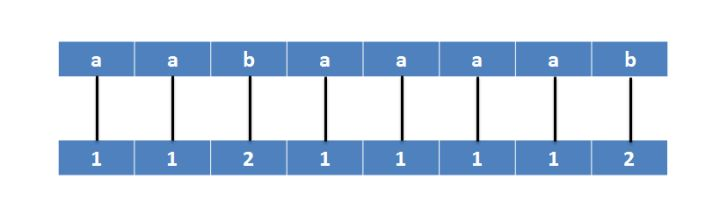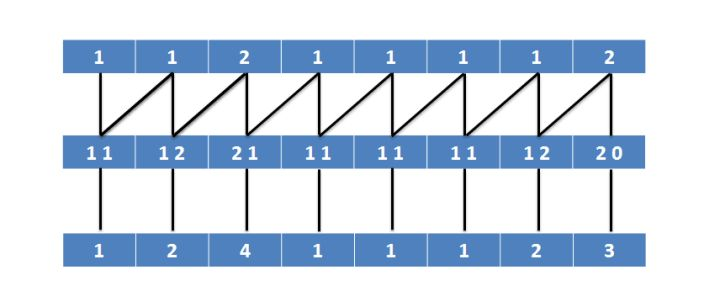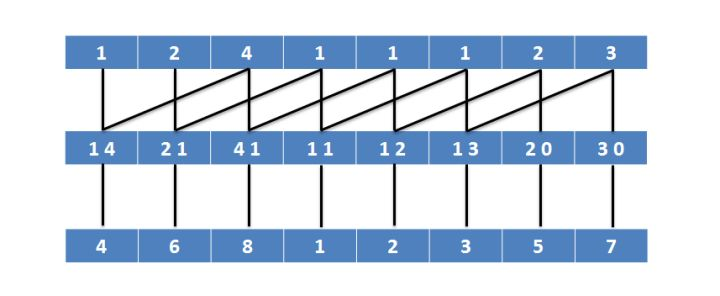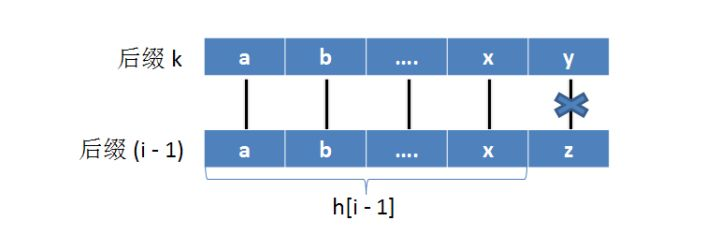# 后缀数组

## 后缀数组的构造1.用vector模拟基数排序，便于理解但常数大

2.每次倍增都更新SA，通过叠加得到名次

## 后缀数组的应用

### 求LCP

$height[i]$表示串$sa[i]$与串$sa[i-1]$的LCP

$h[i]=height[rank[i]]$$rank[k]=rank[i-1]-1$，则$h[i-1]=LCP\{i-1,k\}$

### 求子串Rank

$n-sa[i]+1-height[i]$的前缀和，就能得到比该子串小的个数了